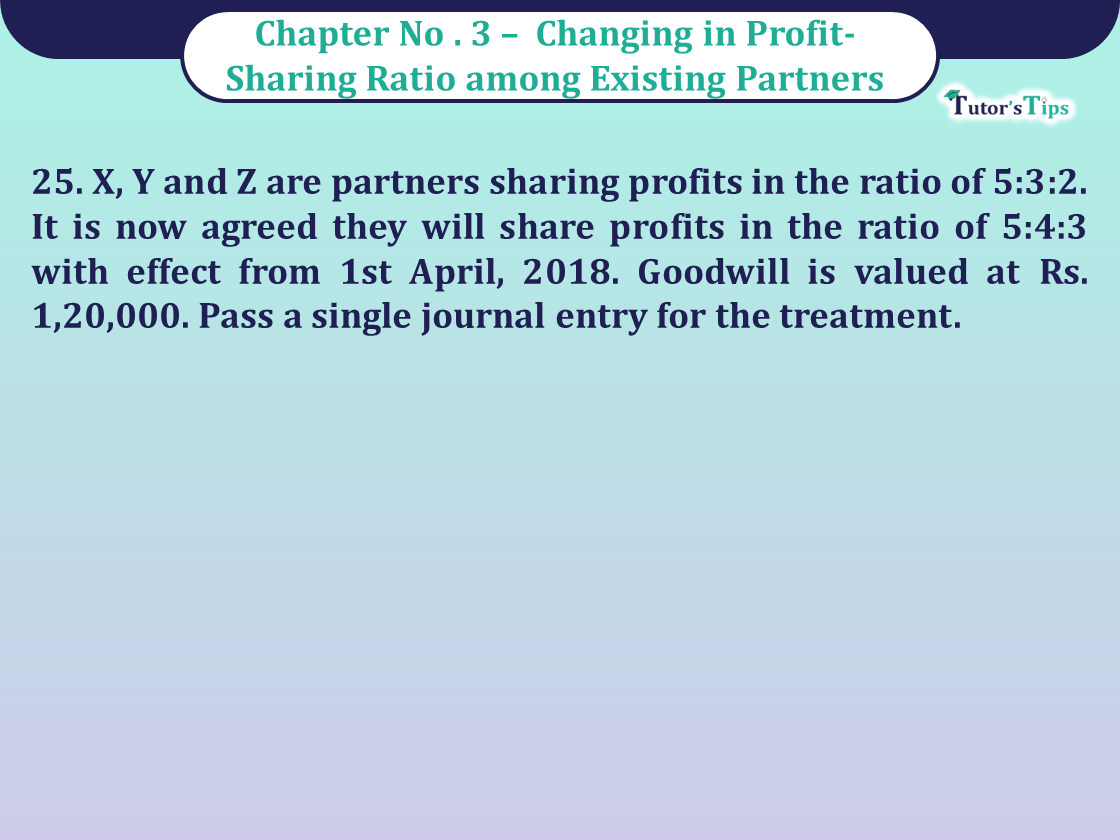# Question 25 Chapter 3 of Class 12 Part – 1 VK PublicationQuestion 25 Chapter 3 of Class 12 Part - 1 VK Publication

Question 25 Chapter 3 of Class 12 Part – 1

25. X, Y and Z are partners sharing profits in the ratio of 5:3:2. It is now agreed they will share profits in the ratio of 5:4:3 with effect from 1st April, 2018. Goodwill is valued at Rs. 1,20,000. Pass a single journal entry for the treatment.

## The solution of Question 25 Chapter 3 of Class 12 Part – 1: –

Journal Entry

 Date Advertisement-X Advertisement-Y Particulars L . F Dr. ₹ Cr. ₹ 2018 Y’s Capital A/c Dr. 4,000 April 1 Z’s Capital A/c Dr. 6,000 To Z’s Capital A/c 10,000 ( Being proportionate share of Goodwill adjusted among partners )

Working Notes:
Old Ratio of X, Y and Z = 5:3:2
New Ratio of X, Y and Z = 5:4:3
Sacrificing Ratio = Old Share – New Share

 X = 5 – 5 10 12
 = 60 – 50 120
 = 10 (Sacrifice) 120

 Y = 3 – 4 10 12
 = 36 – 40 120

 = -4 (Gaining) 20
 Z = 2 – 3 10 12
 = 24 – 30 120
 = -6 (Gaining) 20

Thus Proportionate Share of Goodwill to be adjusted

 X = 1,20,000 X 10 120

= 10,000

 Y = 1,20,000 X 4 120

= 4,000

 Z = 1,20,000 X 6 120

= 6,000

Comment if you have any questions.

Also, Check out the solved question of previous Chapters: –